# Lane Detection System

December 3, 2021

By  Abhishek Tyagi

lang: en_US

## Introduction

It is well known that lane recognition on freeways is an essential part of any successful autonomous driving system. An autonomous car consists of an extensive sensor system and several control modules. The most critical step to robust autonomous driving is to recognize and understand your surroundings. However, it is not enough to identify obstacles and understand the geometry around a vehicle. Camera-based Lane detection can be an essential step towards environmental awareness. It enables the car to be correctly positioned within the lane, which is crucial for every exit and the back lane - planning decision. Therefore, camera-based accurate lane detection with real-time edge detection is vital for autonomous driving and avoiding traffic accidents.

## Functional Principle

MATLAB serves as an image processing tool for the detection of lanes on the road. The following techniques are used for lane detection.
• Canny Edge Detection
• Region of Interest Selection
• Hough Transform Line Detection

### Frame Extraction and Processing

The first step is to import the video file, initialize the variables, and import from the .mat file in code.

clc
close all
clear
%-------------------------Importing the Video File-------------------------
%-----------------Defining Variables for saving Video File-----------------
Output_Video=VideoWriter('Output_video');
Output_Video.FrameRate= 25;
open(Output_Video);

### White and Yellow Image Masking

A color mask isolates a specific color in an image. You can apply a color mask to a clip to correct a particular color, exclude that color from corrections in the rest of the Image, or both. The frame is read first and filtered with a Gaussian filter. The frame is masked with yellow and white so that the lane lines can be seen perfectly.

%% Initializing the loop to take frames one by one
while hasFrame(VideoFile)
%------------------Reading each frame from Video File------------------
% figure('Name','Original Image'), imshow(frame);
frame = imgaussfilt3(frame);
% figure('Name','Filtered Image'), imshow(frame);
%% Masking the image for White and Yellow Color
%--------------Define Thresholds for masking Yellow Color--------------

%----------------------Define thresholds for 'Hue'---------------------
channel1MinY = 130;
channel1MaxY = 255;
%------------------Define thresholds for 'Saturation'------------------
channel2MinY = 130;
channel2MaxY = 255;
%---------------------Define thresholds for 'Value'--------------------
channel3MinY = 0;
channel3MaxY = 130;
%-----------Create mask based on chosen histogram thresholds-----------
Yellow=((frame(:,:,1)>=channel1MinY)|(frame(:,:,1)<=channel1MaxY))& ...
(frame(:,:,2)>=channel2MinY)&(frame(:,:,2)<=channel2MaxY)&...
(frame(:,:,3)>=channel3MinY)&(frame(:,:,3)<=channel3MaxY);
%--------------Define Thresholds for masking White Color---------------

%----------------------Define thresholds for 'Hue'---------------------
channel1MinW = 200;
channel1MaxW = 255;
%------------------Define thresholds for 'Saturation'------------------
channel2MinW = 200;
channel2MaxW = 255;
%---------------------Define thresholds for 'Value'--------------------
channel3MinW = 200;
channel3MaxW = 255;

%-----------Create mask based on chosen histogram thresholds-----------
White=((frame(:,:,1)>=channel1MinW)|(frame(:,:,1)<=channel1MaxW))&...
(frame(:,:,2)>=channel2MinW)&(frame(:,:,2)<=channel2MaxW)& ...
(frame(:,:,3)>=channel3MinW)&(frame(:,:,3)<=channel3MaxW);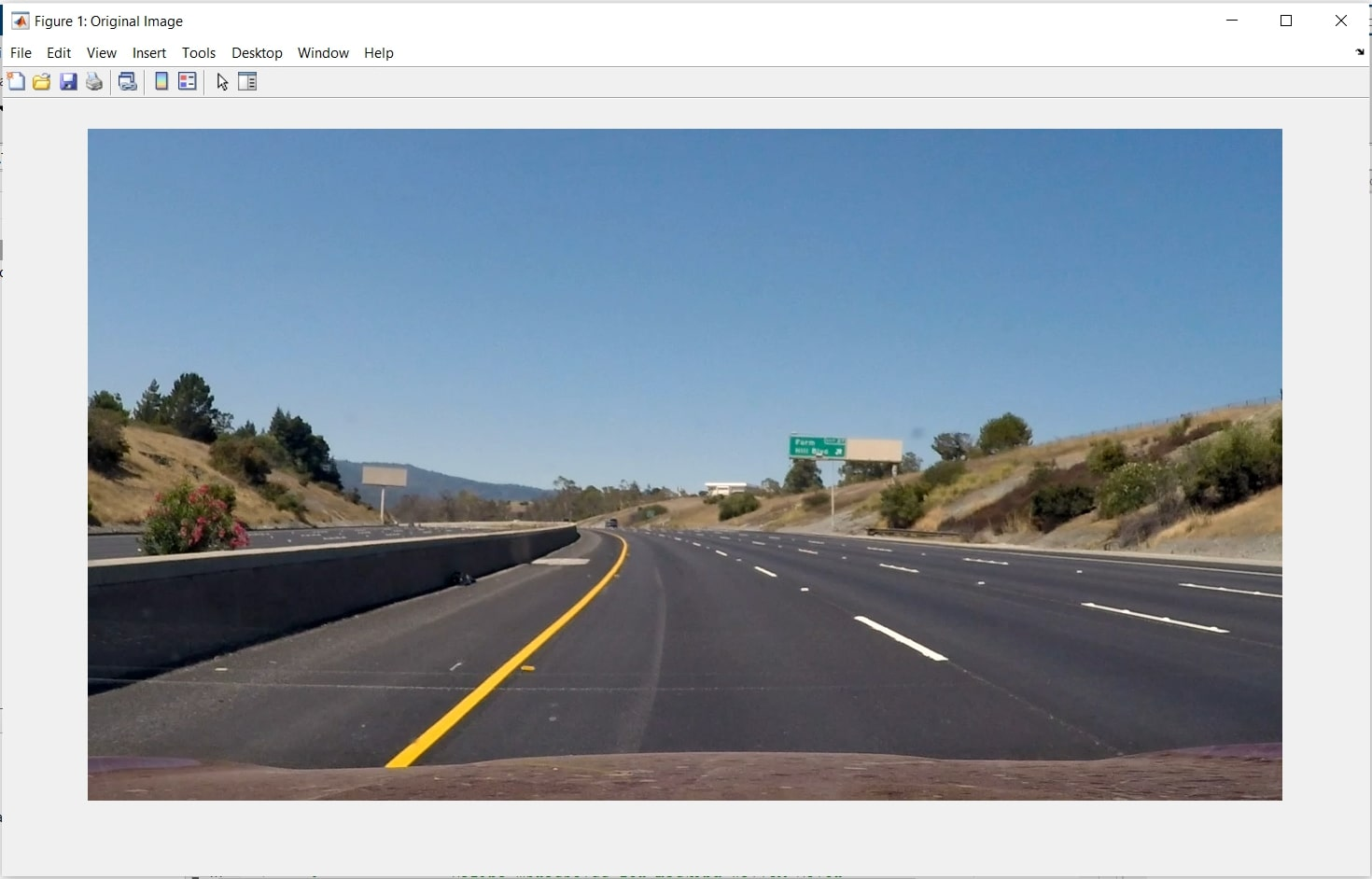Original Image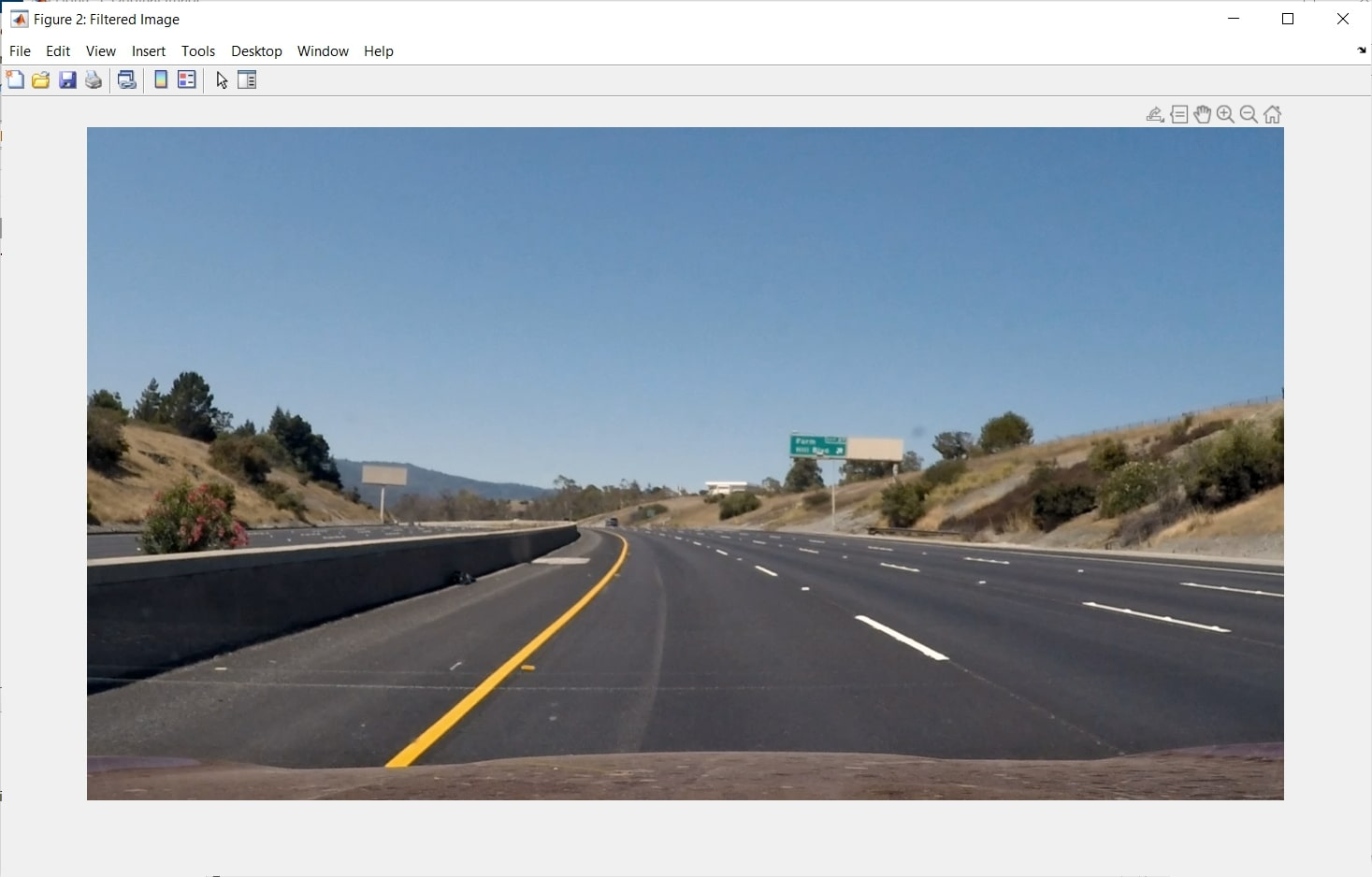Gaussian Filtered Image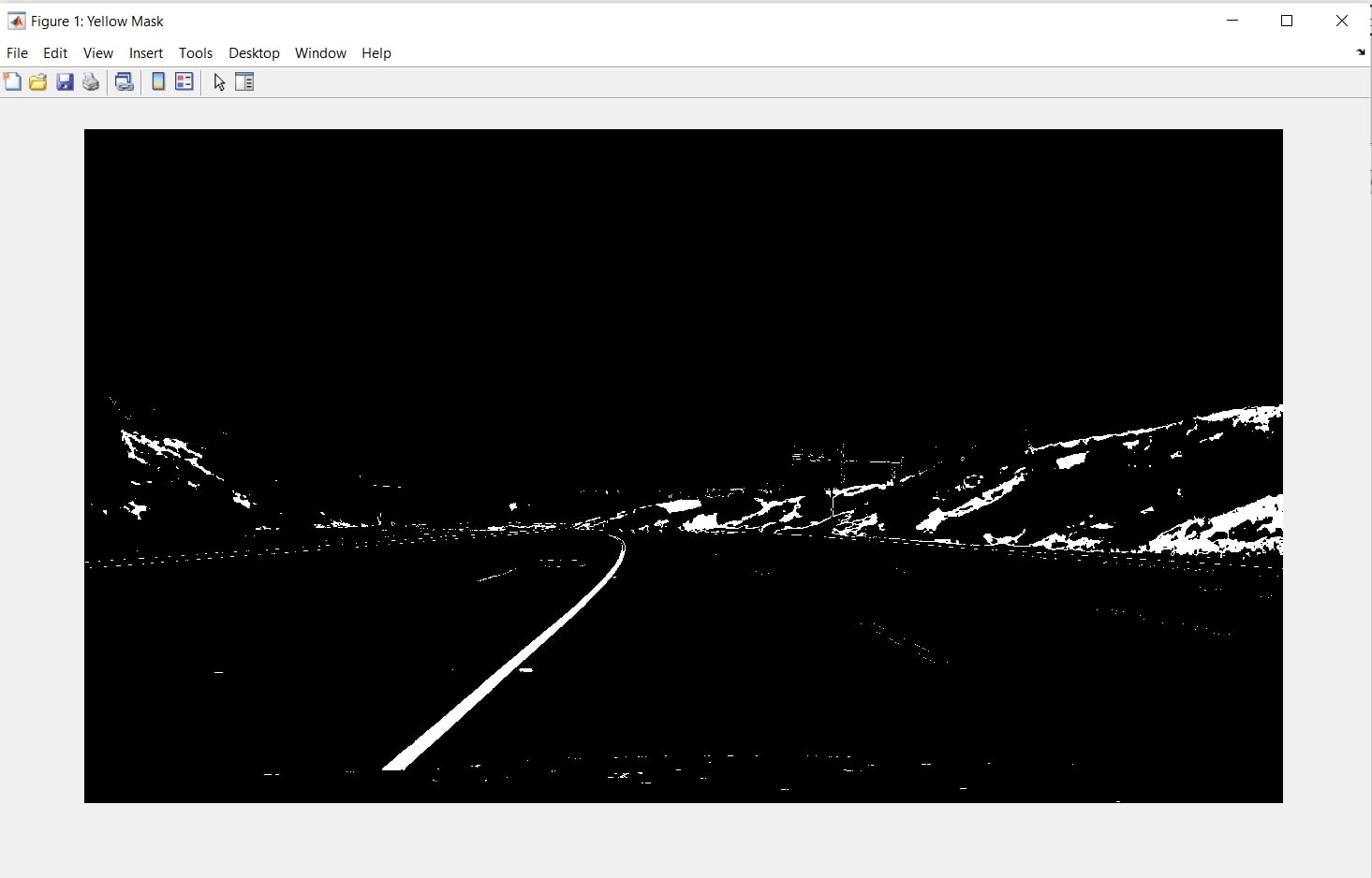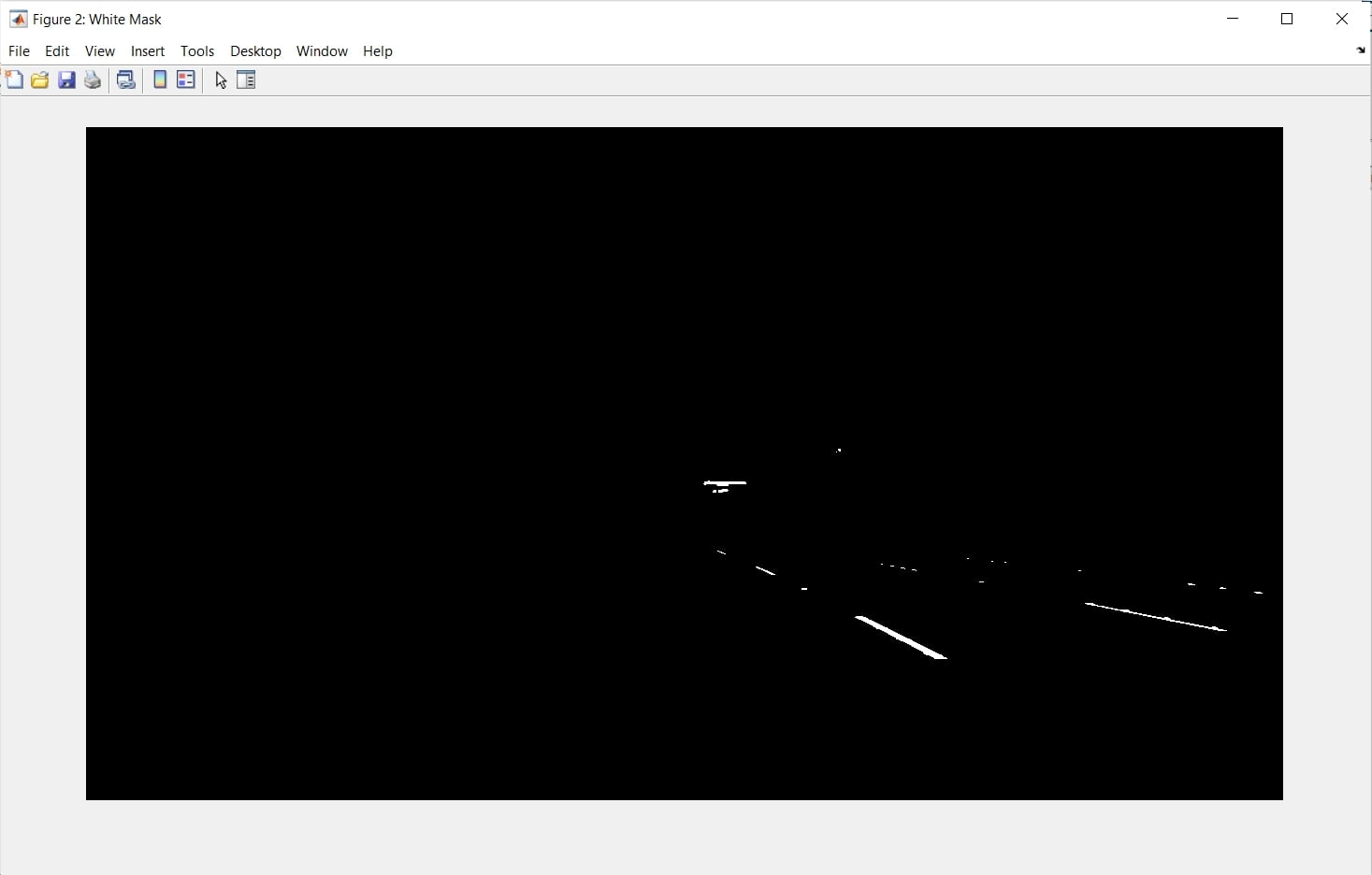### Edge detection

This section extracts edges from the masked Image and neglects closed edges with smaller areas. Edge detection is an image processing technique used to identify points in a digital image with discontinuities. are called the edges (or borders) of the picture.

%% Detecting edges in the image using Canny edge function
frameW = edge(White, 'canny', 0.2);
frameY = edge(Yellow, 'canny', 0.2);

%% Neglecting closed edges in smaller areas
frameY = bwareaopen(frameY,15);
frameW = bwareaopen(frameW,15);
% figure('Name','Detecting Edges of Yellow mask'), imshow(frameY);
% figure('Name','Detecting Edges of White mask'), imshow(frameW);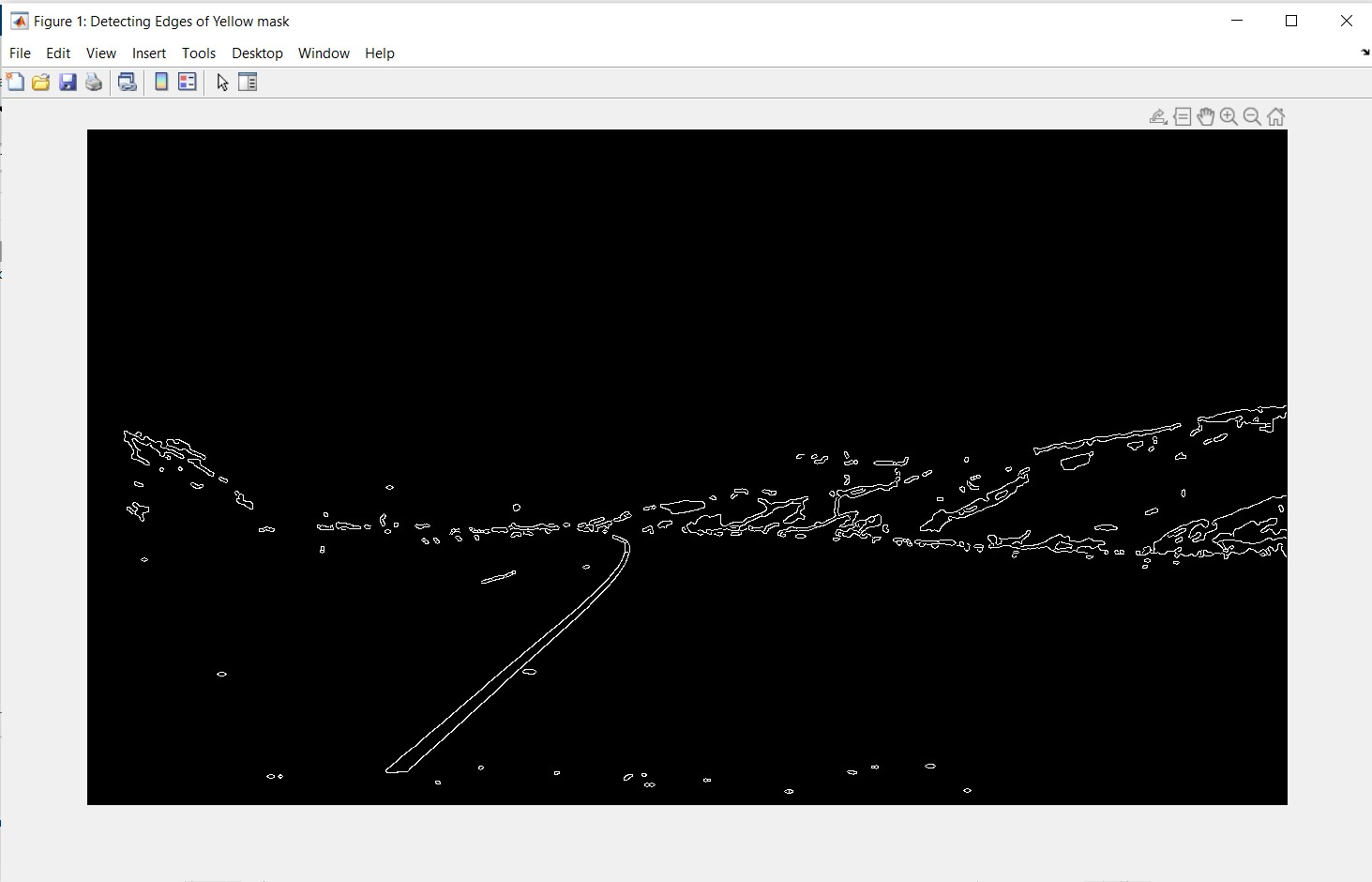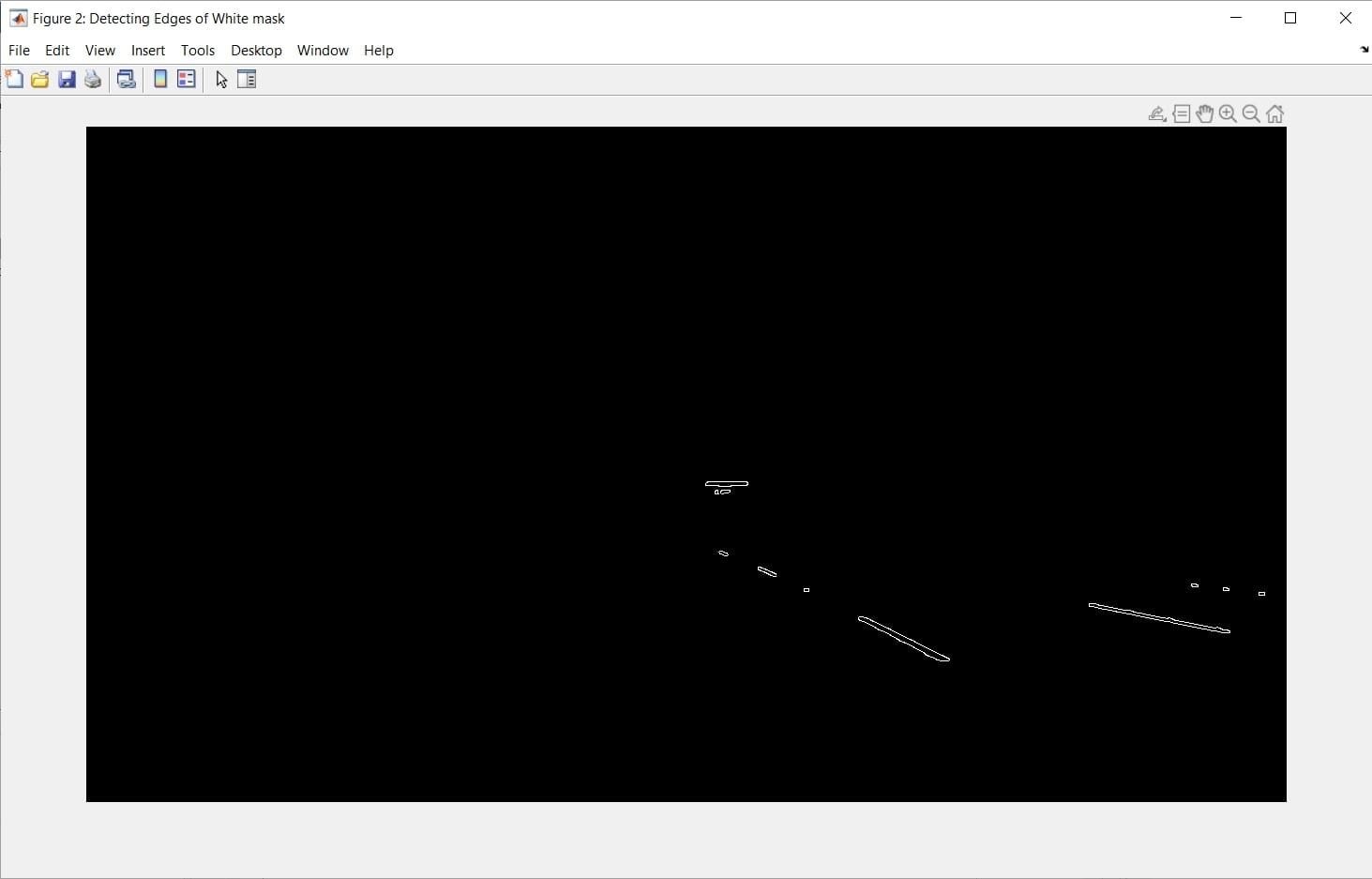### Extraction of the region of interest

As indicated in the pipeline for project implementation, the region of interest is extracted with the function 'Roipoly' and selection of frame points. An area of interest (ROI) is part of an image that you want to filter or edit somehow. You can display an ROI as a binary mask image: In the mask image, the pixels belonging to the ROI are set to 1, and the pixels outside the ROI are set to 0.

%% Extracting ROI
%---------Extracting Region of Interest from Yellow Edge Frame---------
roiY = roipoly(frameY, r, c);
[R , C] = size(roiY);
for i = 1:R
for j = 1:C
if roiY(i,j) == 1
frame_roiY(i,j) = frameY(i,j);
else
frame_roiY(i,j) = 0;
end
end
end
% figure('Name','Filtering ROI from Yellow mask'), imshow(frame_roiY);

%---------Extracting Region of Interest from White Edge Frame----------
roiW = roipoly(frameW, r, c);
[R , C] = size(roiW);
for i = 1:R
for j = 1:C
if roiW(i,j) == 1
frame_roiW(i,j) = frameW(i,j);
else
frame_roiW(i,j) = 0;
end
end
end
% figure('Name','Filtering ROI from White mask'), imshow(frame_roiW);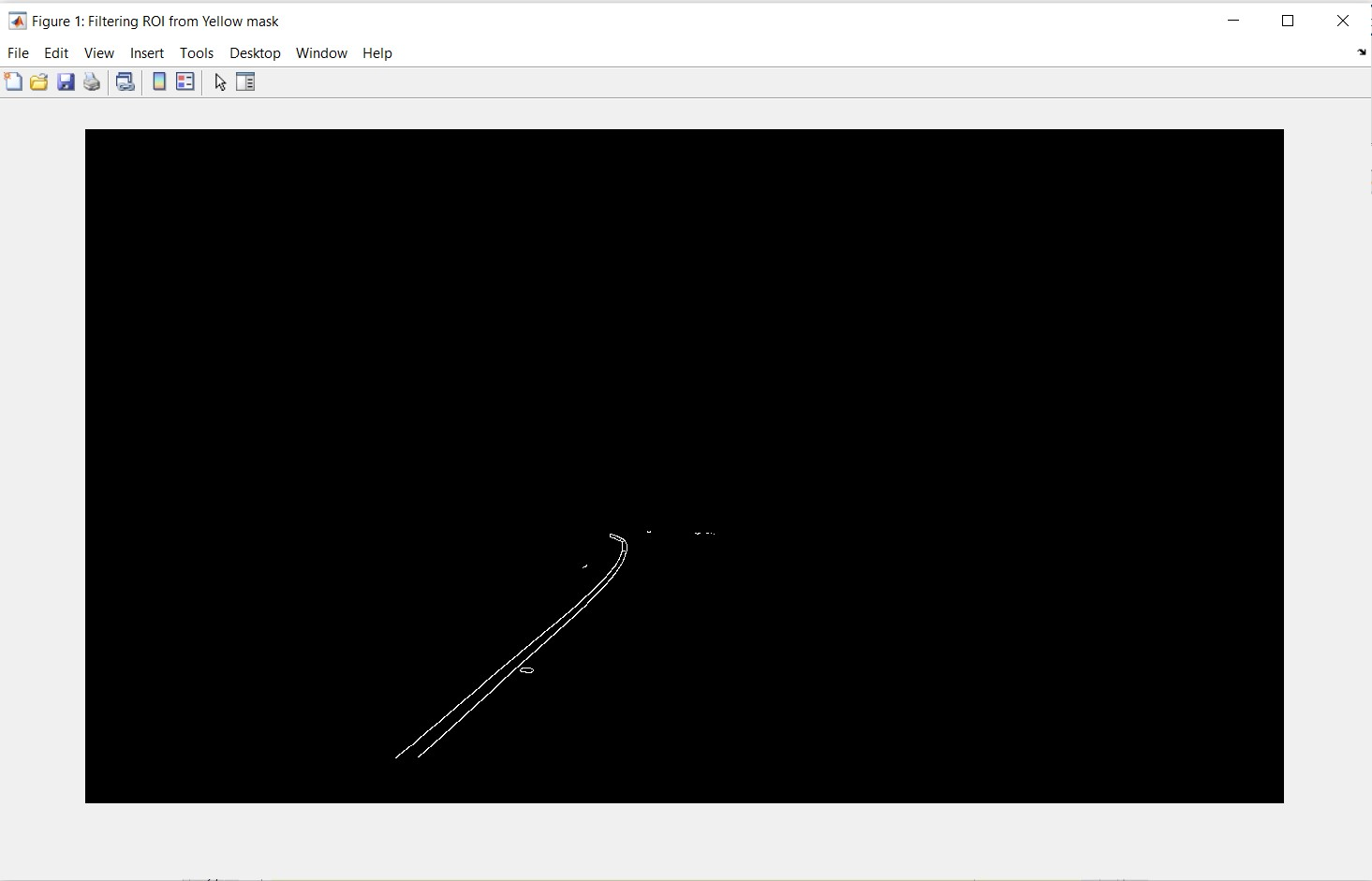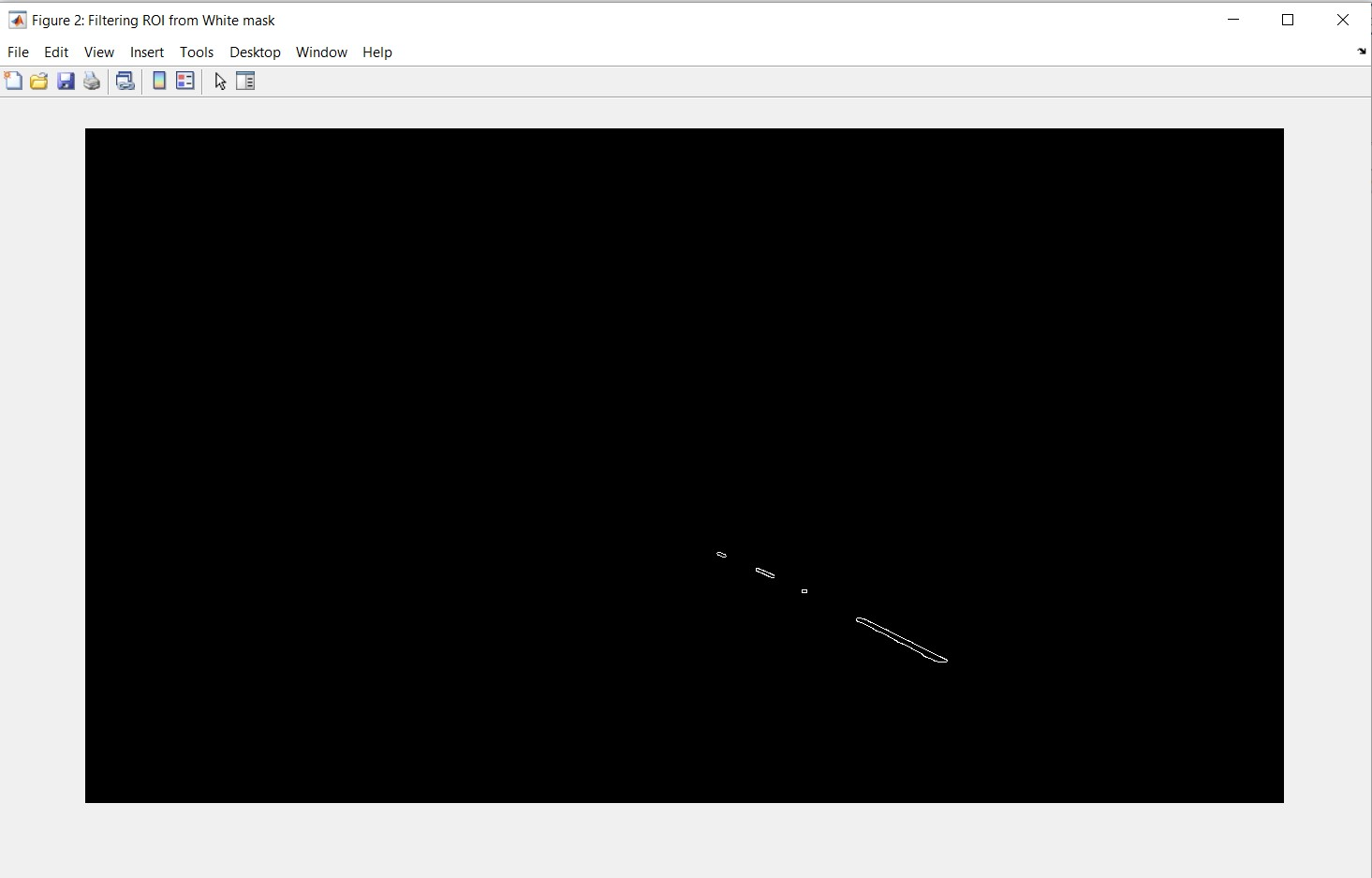### Hough Transform

In this section, I have used the Hough function to get the Hough transform of the binary edge-detected Image that gives us the Hough values. Then I have the Hough diagram. It is a technique that can be used to isolate features of a particular shape within an image since the desired features must be parametrically specified in some way.

%% Applying Hough Tansform to get straight lines from Image

%----------Applying Hough Transform to White and Yellow Frames---------
[H_Y,theta_Y,rho_Y] = hough(frame_roiY);
[H_W,theta_W,rho_W] = hough(frame_roiW);

%--------Extracting Hough Peaks from Hough Transform of frames---------
P_Y = houghpeaks(H_Y,2,'threshold',2);
P_W = houghpeaks(H_W,2,'threshold',2);
%----------Plotting Hough Transform and detecting Hough Peaks----------

% figure('Name','Hough Peaks for White Line')
% xlabel('\theta (degrees)')
% ylabel('\rho')
% axis on
% axis normal
% hold on
% colormap(gca,hot)

% x = theta_W(P_W(:,2));
% y = rho_W(P_W(:,1));
% plot(x,y,'s','color','blue');
% hold off

% figure('Name','Hough Peaks for Yellow Line')
% xlabel('\theta (degrees)')
% ylabel('\rho')
% axis on
% axis normal
% hold on
% colormap(gca,hot)

% x = theta_W(P_Y(:,2));
% y = rho_W(P_Y(:,1));
% plot(x,y,'s','color','blue');
% hold off

%--------------Extracting Lines from Detected Hough Peaks--------------

lines_Y = houghlines(frame_roiY,theta_Y,rho_Y,P_Y,'FillGap',3000,'MinLength',20);
% figure('Name','Hough Lines found in image'), imshow(frame), hold on
% max_len = 0;
% for k = 1:length(lines_Y)
% xy = [lines_Y(k).point1; lines_Y(k).point2];
% plot(xy(:,1),xy(:,2),'LineWidth',2,'Color','green');

% Plot beginnings and ends of lines
% plot(xy(1,1),xy(1,2),'x','LineWidth',2,'Color','yellow');
% plot(xy(2,1),xy(2,2),'x','LineWidth',2,'Color','red');
% end

lines_W = houghlines(frame_roiW,theta_W,rho_W,P_W,'FillGap',3000,'MinLength',20);
max_len = 0;
% for k = 1:2
% xy = [lines_W(k).point1; lines_W(k).point2];
% plot(xy(:,1),xy(:,2),'LineWidth',2,'Color','green');

% Plot beginnings and ends of lines
% plot(xy(1,1),xy(1,2),'x','LineWidth',2,'Color','yellow');
% plot(xy(2,1),xy(2,2),'x','LineWidth',2,'Color','red');
% end
% hold off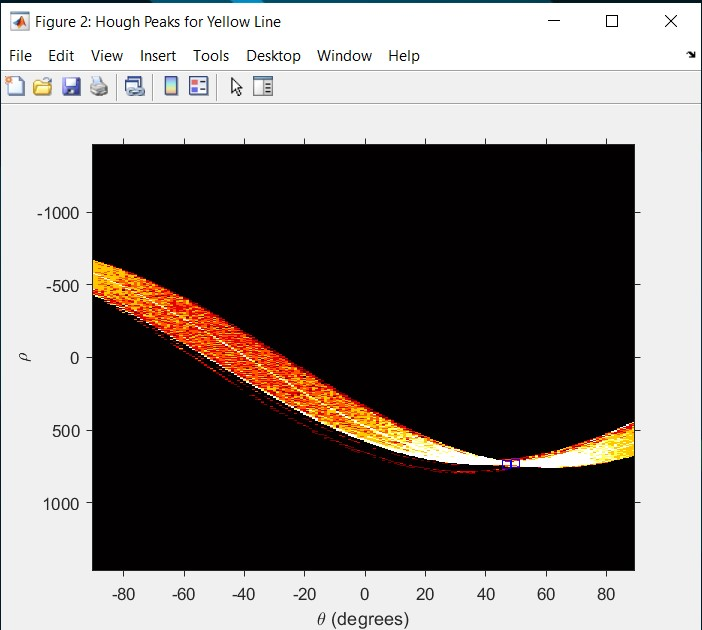Hough peaks found for yellow lines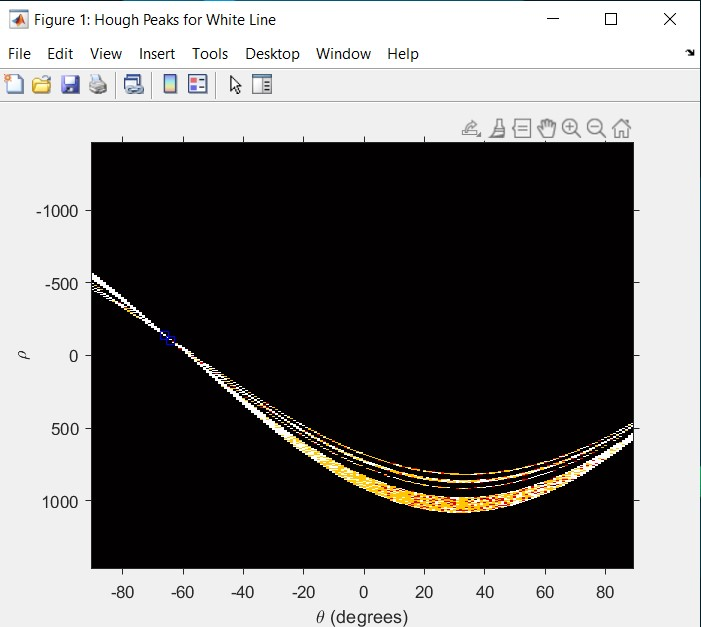Hough peaks found for white lines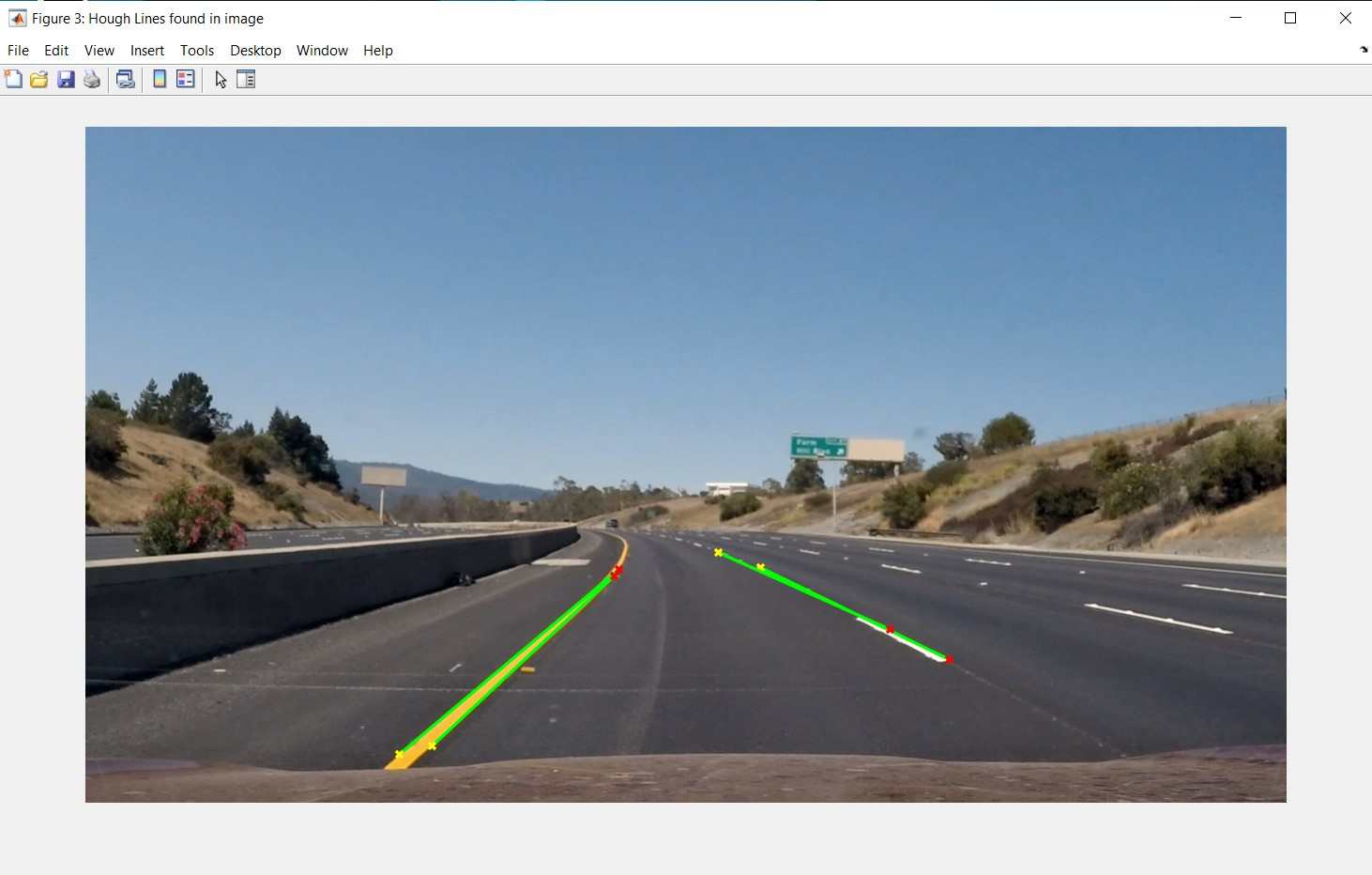Hough lines found in image

### Canny's edge detection algorithm in connection with Hough transform

Canny's edge detection algorithm has the advantage of a higher computational speed. Still, it can overlook some apparent details of the crossing edge because isotropic Gaussian kernels are used.

### Extrapolating lines

In this section, the lines into Hough lines are extrapolated into the filtered main Image.

%% Plotting best fitting line after Extrapolation

%-----------------Extract start and end points of lines----------------

leftp1 = [lines_Y(1).point1; lines_Y(1).point2];
leftp2 = [lines_Y(2).point1; lines_Y(2).point2];

rightp1 = [lines_W(1).point1; lines_W(1).point2];
rightp2 = [lines_W(2).point1; lines_W(2).point2];

if leftp1(1,1) < leftp2(1,1)
left_plot(1,:) = leftp1(1,:);
else
left_plot(1,:) = leftp2(1,:);
end

if leftp1(2,2) < leftp2(2,2)
left_plot(2,:) = leftp1(2,:);
else
left_plot(2,:) = leftp2(2,:);
end

if rightp1(1,2) < rightp2(1,2)
right_plot(1,:) = rightp1(1,:);
else
right_plot(1,:) = rightp2(1,:);
end

if rightp1(2,1) > rightp2(2,2)
right_plot(2,:) = rightp1(2,:);
else
right_plot(2,:) = rightp2(2,:);
end

%----------------Calculate slope of left and right lines---------------

slopeL = (left_plot(2,2)-left_plot(1,2))/(left_plot(2,1)-left_plot(1,1));
slopeR = (right_plot(2,2)-right_plot(1,2))/(right_plot(2,1)-right_plot(1,1));

%------Make equations of left and right lines to extrapolate them------

xLeftY = 1; % x is on the left edge
yLeftY = slopeL * (xLeftY - left_plot(1,1)) + left_plot(1,2);
xRightY = 550; % x is on the reight edge.
yRightY = slopeL * (xRightY - left_plot(2,1)) + left_plot(2,2);

xLeftW = 750; % x is on the left edge
yLeftW = slopeR * (xLeftW - right_plot(1,1)) + right_plot(1,2);
xRightW = 1300; % x is on the reight edge.
yRightW = slopeR * (xRightW - right_plot(2,1)) + right_plot(2,2);

%------Making a transparent Trapezoid between 4 points of 2 lines-------
points = [xLeftY yLeftY; xRightY yRightY ;xLeftW yLeftW; xRightW yRightW ];
number = [1 2 3 4];

### Output Video

After applying various algorithms, we will obtain results after plotting everything on the Image and writing it into video.

%% Plotting Everything on the image

%--Plot the extrapolated lines, Trapezoid and direction on each frame--
%figure('Name','Final Output')
imshow(frame);
hold on
plot([xLeftY, xRightY], [yLeftY, yRightY], 'LineWidth',8,'Color','red');
plot([xLeftW, xRightW], [yLeftW, yRightW], 'LineWidth',8,'Color','red');
patch('Faces', number, 'Vertices', points, 'FaceColor','green','Edgecolor','green','FaceAlpha',0.4)
hold off

%------------------Save each frame to the Output File------------------
writeVideo(Output_Video,getframe);
end
%---------------------Closing Save Video File Variable---------------------
close(Output_Video)Final_image

## Summary

Step 1: Load image or video

Step 2: Frame conversion  The data set has a video format converted into frames for processing.

Step 3: Image Edge Recognition  Algorithm: Canny Edge Recognition

Step 4: Segmentation of the area of interest. After the edge detection by the canny edge detection algorithm, we can see that the edge obtained contains the required lane line edges and other unnecessary lanes and the edges of the surrounding fences. This method can increase the speed and accuracy of the system.

Step 5: Lane recognition Algorithm: Hough Transform Snapshot of the lane recognition output with the Hough transform method.

Step 6: Plot everything on the Image and write it into the output video.

Did you find some helpful content from our video or article and now looking for its code, model, or application? You can purchase the specific Title, if available, and instantly get the download link.

If you are looking for free help, you can post your comment below & wait for any community member to respond, which is not guaranteed. You can book Expert Help, a paid service, and get assistance in your requirement. If your timeline allows, we recommend you book the Research Assistance plan. If you want to get trained in MATLAB or Simulink, you may join one of our training modules.

If you are ready for the paid service, share your requirement with necessary attachments & inform us about any Service preference along with the timeline. Once evaluated, we will revert to you with more details and the next suggested step.

Education is our future. MATLAB is our feature. Happy MATLABing!

#### Abhishek Tyagi

Abhishek Tyagi is an aspiring Automotive engineer who enjoys connecting the dots: be it ideas from different disciplines, people from different teams, or applications from different industries. He has strong technical skills and an academic background in engineering, statistics, and machine learning.

#### Connect with MATLAB Helper ®

Use Website Chat or WhatsApp at +91-8104622179

Watch our Latest Video

Join a Training Module